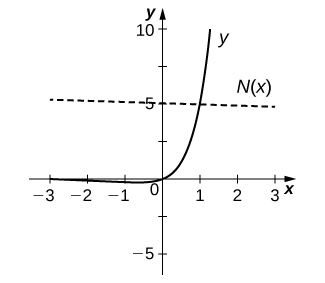$$\newcommand{\id}{\mathrm{id}}$$ $$\newcommand{\Span}{\mathrm{span}}$$ $$\newcommand{\kernel}{\mathrm{null}\,}$$ $$\newcommand{\range}{\mathrm{range}\,}$$ $$\newcommand{\RealPart}{\mathrm{Re}}$$ $$\newcommand{\ImaginaryPart}{\mathrm{Im}}$$ $$\newcommand{\Argument}{\mathrm{Arg}}$$ $$\newcommand{\norm}{\| #1 \|}$$ $$\newcommand{\inner}{\langle #1, #2 \rangle}$$ $$\newcommand{\Span}{\mathrm{span}}$$

# 2.8E: Exercises

$$\newcommand{\vecs}{\overset { \rightharpoonup} {\mathbf{#1}} }$$ $$\newcommand{\vecd}{\overset{-\!-\!\rightharpoonup}{\vphantom{a}\smash {#1}}}$$$$\newcommand{\id}{\mathrm{id}}$$ $$\newcommand{\Span}{\mathrm{span}}$$ $$\newcommand{\kernel}{\mathrm{null}\,}$$ $$\newcommand{\range}{\mathrm{range}\,}$$ $$\newcommand{\RealPart}{\mathrm{Re}}$$ $$\newcommand{\ImaginaryPart}{\mathrm{Im}}$$ $$\newcommand{\Argument}{\mathrm{Arg}}$$ $$\newcommand{\norm}{\| #1 \|}$$ $$\newcommand{\inner}{\langle #1, #2 \rangle}$$ $$\newcommand{\Span}{\mathrm{span}}$$ $$\newcommand{\id}{\mathrm{id}}$$ $$\newcommand{\Span}{\mathrm{span}}$$ $$\newcommand{\kernel}{\mathrm{null}\,}$$ $$\newcommand{\range}{\mathrm{range}\,}$$ $$\newcommand{\RealPart}{\mathrm{Re}}$$ $$\newcommand{\ImaginaryPart}{\mathrm{Im}}$$ $$\newcommand{\Argument}{\mathrm{Arg}}$$ $$\newcommand{\norm}{\| #1 \|}$$ $$\newcommand{\inner}{\langle #1, #2 \rangle}$$ $$\newcommand{\Span}{\mathrm{span}}$$

## Exercise $$\PageIndex{1}$$

For the following exercises, find $$f′(x)$$ for each function.

1) $$f(x)=x^2e^x$$

$$2xe^x+x^2e^x$$

2) $$f(x)=\frac{e^{−x}}{x}$$

3) $$f(x)=e^{x^3lnx}$$

$$e^{x^3}lnx(3x^2lnx+x^2)$$

4) $$f(x)=\sqrt{e^{2x}+2x}$$

5) $$f(x)=\frac{e^x−e^{−x}}{e^x+e^{−x}}$$

$$\frac{4}{(e^x+e^{−x})^2}$$

6) $$f(x)=\frac{10^x}{ln10}$$

7) $$f(x)=2^{4x}+4x^2$$

$$2^{4x+2}⋅ln2+8x$$

8) $$f(x)=3^{sin3x}$$

9) $$f(x)=x^π⋅π^x$$

$$πx^{π−1}⋅π^x+x^π⋅π^xlnπ$$

10) $$f(x)=ln(4x^3+x)$$

11) $$f(x)=ln\sqrt{5x−7}$$

$$\frac{5}{2(5x−7)}$$

12) $$f(x)=x^2ln9x$$

13) $$f(x)=log(secx)$$

$$\frac{tanx}{ln10}$$

14) $$f(x)=log_7(6x^4+3)^5$$

15) $$f(x)=2^x⋅log_37^{x^2−4}$$

$$2^x⋅ln2⋅log_37^{x^2−4}+2^x⋅\frac{2xln7}{ln3}$$

## Exercise $$\PageIndex{2}$$

For the following exercises, use logarithmic differentiation to find $$\frac{dy}{dx}$$.

1) $$y=x^{\sqrt{x}}$$

2) $$y=(sin2x)^{4x}$$

$$(sin2x)^{4x}[4⋅ln(sin2x)+8x⋅cot2x]$$

3) $$y=(lnx)^{lnx}$$

4) $$y=x^{log_2x}$$

$$x^{log_2x}⋅\frac{2lnx}{xln2}$$

5) $$y=(x^2−1)^{lnx}$$

6) $$y=x^{cotx}$$

$$x^{cotx}⋅[−csc^2x⋅lnx+\frac{cotx}{x}]$$

7) $$y=\frac{x+11}{\sqrt{x^2−4}}$$

8) $$y=x^{−1/2}(x^2+3)^{2/3}(3x−4)^4$$

$$x^{−1/2}(x2+3)^{2/3}(3x−4)^4⋅[\frac{−1}{2x}+\frac{4x}{3(x^2+3)}+\frac{12}{3x−4}]$$

## Exercise $$\PageIndex{3}$$

Find an equation of the tangent line to the graph of $$f(x)=4xe^{(x^2−1)}$$ at the point where

$$x=−1.$$ Graph both the function and the tangent line.

Under Construction

## Exercise $$\PageIndex{4}$$

Find the equation of the line that is normal to the graph of $$f(x)=x⋅5^x$$ at the point where $$x=1$$. Graph both the function and the normal line.

$$y=\frac{−1}{5+5ln5}x+(5+\frac{1}{5+5ln5})$$## Exercise $$\PageIndex{5}$$

Find the equation of the tangent line to the graph of $$x^3−xlny+y^3=2x+5$$ at the point where $$x=2$$. Graph both the curve and the tangent line.

(Hint: Use implicit differentiation to find $$\frac{dy}{dx}$$.)

Under Construction

## Exercise $$\PageIndex{6}$$

Consider the function $$y=x^{1/x}$$ for $$x>0.$$

a. Determine the points on the graph where the tangent line is horizontal.

b. Determine the points on the graph where $$y′>0$$ and those where $$y′<0$$.

Solution: $$a. x=e~2.718$$ $$b. (e,∞),(0,e)$$

a. $$x=e~2.718$$

b. $$(e,∞),(0,e)$$

## Exercise $$\PageIndex{7}$$

The formula $$I(t)=\frac{sint}{e^t}$$ is the formula for a decaying alternating current.

a. Complete the following table with the appropriate values.

 $$t$$ $$\frac{sint}{e^t}$$ 0 (i) $$π/2$$ (ii) $$π$$ (iii) $$3π/2$$ (vi) $$2π$$ (v) $$2π$$ (vi) $$3π$$ (vii) $$7π/2$$ (viii) $$4π$$ (ix)

b. Using only the values in the table, determine where the tangent line to the graph of (I(t)\) is horizontal.

Under Construction

## Exercise $$\PageIndex{8}$$

The population of Toledo, Ohio, in 2000 was approximately 500,000. Assume the population is increasing at a rate of 5% per year.

a. Write the exponential function that relates the total population as a function of $$t$$.

b. Use a. to determine the rate at which the population is increasing in $$t$$ years.

c. Use b. to determine the rate at which the population is increasing in 10 years

a. $$P=500,000(1.05)^t$$ individuals

b. $$P′(t)=24395⋅(1.05)^t$$ individuals per year

c. $$39,737$$ individuals per year

## Exercise $$\PageIndex{9}$$

An isotope of the element erbium has a half-life of approximately 12 hours. Initially there are 9 grams of the isotope present.

a. Write the exponential function that relates the amount of substance remaining as a function of $$t$$, measured in hours.

b. Use a. to determine the rate at which the substance is decaying in $$t$$ hours.

c. Use b. to determine the rate of decay at $$t=4$$ hours.

Under Construction

## Exercise $$\PageIndex{10}$$

The number of cases of influenza in New York City from the beginning of 1960 to the beginning of 1961 is modeled by the function

$$N(t)=5.3e^{0.093t^2−0.87t},(0≤t≤4)$$,

where $$N(t)$$ gives the number of cases (in thousands) and t is measured in years, with $$t=0$$ corresponding to the beginning of 1960.

a. Show work that evaluates $$N(0)$$ and $$N(4)$$. Briefly describe what these values indicate about the disease in New York City.

b. Show work that evaluates $$N′(0)$$ and $$N′(3)$$. Briefly describe what these values indicate about the disease in the United States.

a. At the beginning of 1960 there were 5.3 thousand cases of the disease in New York City. At the beginning of 1963 there were approximately 723 cases of the disease in the United States.

b. At the beginning of 1960 the number of cases of the disease was decreasing at rate of $$−4.611$$ thousand per year; at the beginning of 1963, the number of cases of the disease was decreasing at a rate of $$−0.2808$$ thousand per year.

## Exercise $$\PageIndex{11}$$

The relative rate of change of a differentiable function $$y=f(x)$$ is given by $$\frac{100⋅f′(x)}{f(x)}%.$$ One model for population growth is a Gompertz growth function, given by $$P(x)=ae^{−b⋅e^{−cx}}$$ where $$a,b$$, and $$c$$ are constants.

a. Find the relative rate of change formula for the generic Gompertz function.

b. Use a. to find the relative rate of change of a population in $$x=20$$ months when $$a=204,b=0.0198,$$ and $$c=0.15.$$

c. Briefly interpret what the result of b. means.

Under Construction

## Exercise $$\PageIndex{12}$$

For the following exercises, use the population of New York City from 1790 to 1860, given in the following table.

 Year since 1790 Population 0 33,131 10 60,515 20 96,373 30 123,706 40 202,300 50 312,710 60 515,547 70 813,669

New York City Population Over TimeSource: http://en.wikipedia.org/wiki/Largest..._United_States

1) Using a computer program or a calculator, fit a growth curve to the data of the form $$p=ab^t$$.

$$p=35741(1.045)^t$$

2) Using the exponential best fit for the data, write a table containing the derivatives evaluated at each year.

3) Using the exponential best fit for the data, write a table containing the second derivatives evaluated at each year.